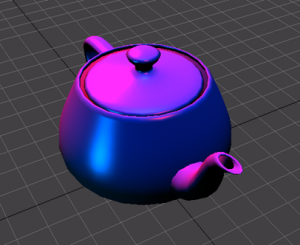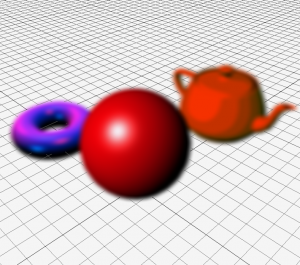Alex Lemke

My Online Portfolio

Time for post process effects!

I started out with a simple black and white post process shader.

Basically it turns thisThis is done by getting the sum of the rgb pixel values and dividing it by three, giving you the grey scale value of that color.Next we have a sobel edge detection post process.  This simply gets the gradient of each pixel(or the change in color) from the surrounding nearby pixels.  If the gradient beats a threshold value then it’s an edge. Also in this image is a basic cel shading effect which is done by rounding the calculated diffuse levels to certain thresholds.Last we have a blur post process effect.  This simply samples the surrounding pixels around the current pixel, scales them depending on their distance from the current pixel and then adds up the calculated values.  This shader is done in two passes, first blurring the horizontally then vertically.  All of these shaders were done in FxComposer.

Post Process Black and White Effect

Post Process Sobel Edge Detection